# python 进程(process)阻塞

import time
import multiprocessing

def worker(name):
print('%s: %s start...' % (time.strftime('%X'), name))
time.sleep(2)
print('%s: %s done.' % (time.strftime('%X'), name))

def xxx():
pass

if __name__ == '__main__':
xxx()

def 单进程阻塞():
t = multiprocessing.Process(target=worker, args=('张三',))
t.start()
# 阻塞
t.join()
print('Finished')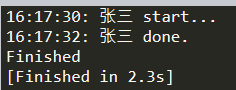def 单进程不阻塞():
t = multiprocessing.Process(target=worker, args=('李四',))
t.start()
print('Finished')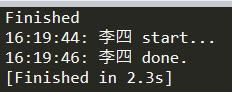def 多进程的错误阻塞():
t1 = multiprocessing.Process(target=worker, args=('张三',))
t1.start()
t1.join()
t2 = multiprocessing.Process(target=worker, args=('李四',))
t2.start()
t2.join()
print('Finished')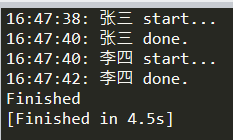def 多进程的正确阻塞():
t1 = multiprocessing.Process(target=worker, args=('张三',))
t2 = multiprocessing.Process(target=worker, args=('李四',))
t1.start()
t2.start()
t1.join()
t2.join()
print('Finished')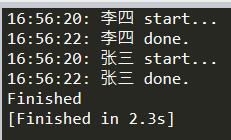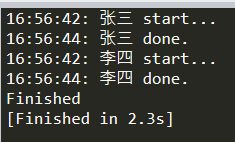def worker(name):
print('%s: %s start...' % (time.strftime('%X'), name))
time.sleep(2 if name == '张三' else 1)
print('%s: %s done.' % (time.strftime('%X'), name))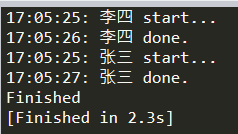def 多进程不阻塞():
t1 = multiprocessing.Process(target=worker, args=('张三',))
t2 = multiprocessing.Process(target=worker, args=('李四',))
t1.start()
t2.start()
print('Finished')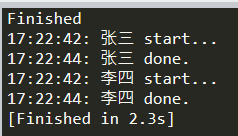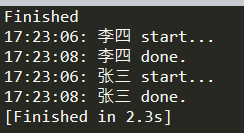def 守护进程阻塞():
t1 = multiprocessing.Process(daemon=True, target=worker, args=('张三',))
t2 = multiprocessing.Process(daemon=True, target=worker, args=('李四',))
t1.start()
t2.start()
t1.join()
t2.join()
print('Finished')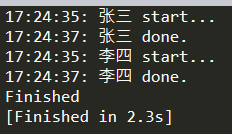def 守护进程不阻塞():
t1 = multiprocessing.Process(daemon=True, target=worker, args=('张三',))
t2 = multiprocessing.Process(daemon=True, target=worker, args=('李四',))
t1.start()
t2.start()
print('Finished')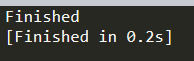def 守护进程不阻塞但主进程比较晚结束():
t1 = multiprocessing.Process(daemon=True, target=worker, args=('张三',))
t2 = multiprocessing.Process(daemon=True, target=worker, args=('李四',))
t1.start()
t2.start()
time.sleep(5)
print('Finished')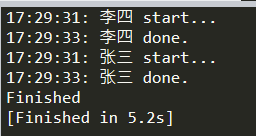def 进程池阻塞():
with multiprocessing.Pool(processes=3) as pp:
pp.apply(worker, ('张三',))
pp.apply(worker, ('李四',))
pp.close()
pp.join()
print('Finished')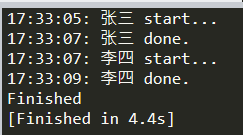def 进程池不阻塞():
with multiprocessing.Pool(processes=3) as pp:
pp.apply(worker, ('张三',))
pp.apply(worker, ('李四',))
pp.close()
print('Finished')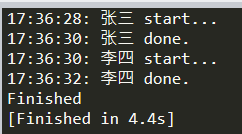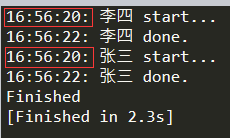与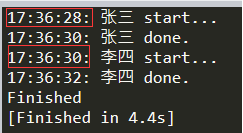def 进程池异步阻塞():
with multiprocessing.Pool(processes=3) as pp:
pp.apply_async(worker, ('张三',))
pp.apply_async(worker, ('李四',))
pp.close()
pp.join()
print('Finished')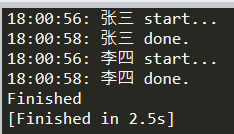def 进程池异步不阻塞():
with multiprocessing.Pool(processes=3) as pp:
pp.apply_async(worker, ('张三',))
pp.apply_async(worker, ('李四',))
pp.close()
print('Finished')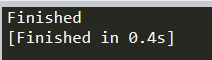def 进程池同步并发不阻塞():
with multiprocessing.Pool(processes=3) as pp:
pp.map(worker, ['张三', '李四'])
pp.close()
print('Finished')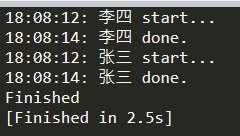def 进程池同步并发阻塞():
with multiprocessing.Pool(processes=3) as pp:
pp.map(worker, ['张三', '李四'])
pp.map(worker, ['王五', '赵六'])
pp.close()
print('Finished')posted @ 2020-09-17 18:19  Tiac  阅读(2628)  评论(0编辑  收藏  举报